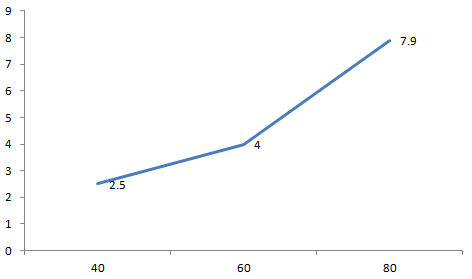# CAT 2001 Question Paper

Instructions

Directions for the following two questions: Answer the questions based on the following information.

The batting average (BA) of a Test batsman is computed from runs scored and innings played — completed innings and incomplete innings (not out) in the following manner:

$$r_1 =$$ Number of runs scored in completed innings

$$n_1 =$$ Number of completed innings

$$r_2 =$$ Number of runs scored in incomplete innings

$$n_2 =$$ Number of incomplete innings

$$BA=\frac{r_1+r_2}{n_1}$$

To better assess a batsman’s accomplishments, the ICC is considering two other measures $$MBA_1$$ and $$MBA_2$$ defined as follows:

$$MBA_1 = \frac{r_1}{n_1} + \frac{n_2}{n_1}*max[0,(\frac{r_2}{n_2}-\frac{r_1}{n_1})]$$

$$MBA_2 = \frac{r_1 + r_2}{n_1 + n_2}$$

Question 1

Question 2

# An experienced cricketer with no incomplete innings has BA of 50. The next time he bats, the innings is incomplete and he scores 45 runs. It can be inferred that

Instructions

Directions for the following two questions: Answer the questions based on the following information.

The petrol consumption rate of a new model car ‘Palto’ depends on its speed and may be described by the graph below.

The axis represents the speed and the Y axis represents the Fuel Consumption (Liters per hour)Question 3

Question 4

# Manasa would like to minimize the fuel consumption for the trip by driving at the appropriate speed. How should she change the speed?[CAT 2001]

Instructions

For the following questions answer them individually

Question 5

Question 6

Question 7

Question 8

Question 9

Question 10

OR